# What if the null hypothesis were true?

## Hypothesis test fully explained

In this article we explain the complete procedure for hypothesis testing. We go into the following topics & steps:

12 tasks with solutions PDF download ✓ preparing for Abiˈ20 ✓

1,99€

### Hypothesis test introduction

Hypothesis tests are always carried out when you want to prove something with the help of collected data, for example that the beer mugs are not completely full at the Oktoberfest. The principle behind all statistical tests is that we have to refute the opposite - we have to refute that the beer mug is actually filled with one liter.

We can imagine this principle with a court hearing, because as the saying goes: In case of doubt, for the accused. The accused is presumed to be innocent (without knowing exactly). In order to be convinced of the guilt of the accused, sufficient evidence must be gathered to demonstrate the guilt beyond a doubt. If there is not enough evidence, he must be presumed to be innocent. We can summarize this fact in statistical hypotheses:

• \$ H_0 \$: The defendant is innocent.
• \$ H_1 \$: The defendant is guilty.

There are thus two contradicting assertions / assumptions (so-called hypotheses).

The null hypothesis \$ H_0 \$ to be tested and its logical negation, the alternative or counter hypothesis \$ H_1 \$. The terms are to be understood in such a way that a check is carried out to determine whether \$ H_1 \$ can be proven, i.e. if the search is unsuccessful, \$ H_0 \$ is still considered valid.

The hypothesis test is used to come to a decision based on the results of a sample as to which of the two hypotheses one is more willing to believe or, in other words: which of the two hypotheses is accepted (or retained) and which is rejected.

Naturally, the hypothesis test can never offer a 100% certainty that the assumed hypothesis is actually true, since we deduce the population from a sample.

The binomial distribution is used to calculate the probabilities of such a test.

In this section we want to give you a rough overview of the topic of testing. Let the statement be: 30% love math, which should be our \$ H_0 \$ hypothesis. With the help of the sample size \$ n = 100 \$ (100 students were interviewed) and the probability \$ p = 0.3 \$, the probability distribution can be represented. The highest value of the distribution always corresponds to the expected value.

The following figure shows us which test should be carried out as a result of the task and what the counter-hypothesis \$ H_1 \$ must be.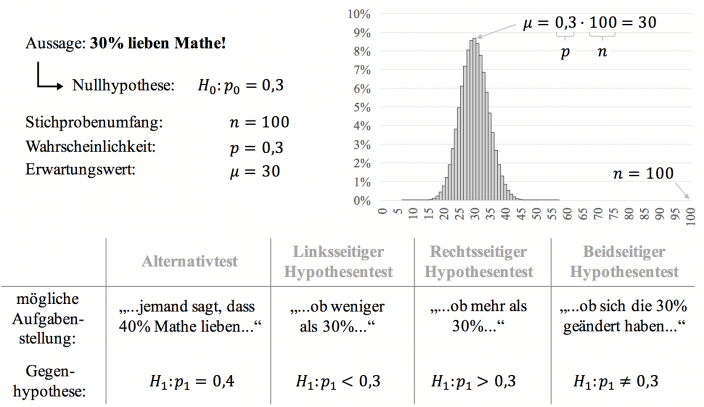If someone claims otherwise, for example that 40% love math instead of 30%, an alternative test is carried out. In addition to the alternative test, there is also the one-sided hypothesis test, which can be left or right-sided, and the two-sided hypothesis test.

In the next few sections we will see how such hypotheses are made, what these errors are all about, and how we perform hypothesis tests with \$ \ sigma \$ rules and the normal distribution table.

12 tasks with solutions PDF download ✓ preparing for Abiˈ20 ✓

1,99€

### Establishing the hypotheses

Before performing a hypothesis test, the hypotheses must be determined.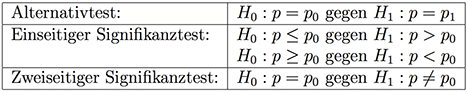How do you know what the \$ H_0 \$ hypothesis and what the \$ H_1 \$ hypothesis is? Note for the significance test (does not apply to alternative tests):

1. In the \$ H_1 \$ hypothesis there is never a =, ≤ or ≥.
2. If the word at most (≤) appears in the exercise, then we know that this characterizes the \$ H_0 \$ hypothesis and that we are performing a right-hand hypothesis test.
3. If the word at least (≥) appears in the problem, then we know that this marks the \$ H_0 \$ hypothesis and we are performing a left-hand hypothesis test.
4. If the word more than or greater than (>) appears in the exercise, then we know that this marks the \$ H_1 \$ hypothesis and that we are performing a right-hand hypothesis test.
5. If the word less than or less (<) appears in the exercise, then we know that this marks the \$ H_1 \$ hypothesis and that we are performing a left-sided hypothesis test.

### Example:

One would like to prove with a test that career starters with a master’s degree earn more on average than career starters with a bachelor’s degree. To this end, 100 young professionals are asked about their degree and starting salary.

What is the null or alternative hypothesis in this case?

Solution:

Since we want to prove that career starters with a master’s degree have a higher starting salary, this assertion must be included in the alternative hypothesis. The null hypothesis is the exact opposite of this. As long as we don't show a difference in income, we have to assume that both groups earn the same:

• \$ H_0 \$: Bachelor and Master graduates receive the same starting salary.
• \$ H_1 \$: Master’s graduates receive a higher starting salary than Bachelor’s graduates.

In mathematical terms: \$ H_0: \ \ mu_M = \ mu_B \$ and \$ H_1: \ \ mu_M> \ mu_B \$ with \$ \ mu_M \$ or \$ \ mu_B \$ as the average starting salary of a master's or bachelor's student.

Watch Daniel's tutorial on hypothesis testing for more in-depth information.

Overview, testing, alternative test, hypothesis test, one-sided, two-sided, math by Daniel Jung

12 tasks + solutions ✓ PDF available immediately ✓ preparing for Abiˈ21 ✓

New!

### Test size and sample length

In order to be able to decide which of the two hypotheses should be accepted and which should be rejected, a random sample is planned. This means that the random experiment in question is repeated \$ n \$ times independently of one another. For example, a survey asks 100 people whether they love math or not. The number of repetitions is called the length of the sample.

What is paid attention to when carrying out the individual tests (i.e. the number of occurrences of the relevant event) is called the test size or test statistic. It is sometimes abbreviated with \$ T \$, often with \$ X \$ or \$ Z \$.

The sample is a Bernoulli chain. The test variable is therefore binomially distributed.

### example

In order to carry out a test, Mr. Jung instructs the employee of his company who is entrusted with the operation of the video camera to watch for the next 100 instructional videos filmed by the camera, how many of them have a bad sound, and to inform him of the result at the end of the week. In this case, what is the sample length and what is the test size in this sample?

Solution:

The sample length is \$ n = \$ 100, and the test size is the number of videos with poor sound among the 100 videos in the sample.

12 tasks with solutions PDF download ✓ preparing for Abiˈ20 ✓

1,99€

### Decision rule: acceptance and rejection area

The hypotheses have been established, the sample has been carried out and we have determined the test size. In order to come to a final decision, we set an acceptance and rejection area. Depending on the value that the test variable assumes in the sample, one will assume the correctness of one or the other of the two hypotheses.

The acceptance range \$ A \$ thus includes the values ​​between \$ 0 \$ and \$ n \$, for which \$ H_0 \$ should be accepted. In contrast to this, the rejection range \$ \ bar {A} \$ includes the other values ​​for which \$ H_0 \$ should be rejected or discarded.

If from Establish decision-making rule is spoken, should be determined for one of the two hypotheses - usually for the null hypothesis - acceptance and rejection range. The decision rule should not be made arbitrarily or by feeling. Because then it can happen that hypotheses are accepted or rejected too easily, which makes the test less meaningful. Therefore, a significance level \$ \ alpha \$ is set beforehand, which ensures the validity of the test.

The following figure shows us what the rejection and acceptance areas look like for the different test types. For the rejection area one always first determines the critical value \$ k \$, which is usually dependent on \$ \ alpha \$, and then specifies the areas. How this critical value can be calculated can be seen in the chapters on the respective test types.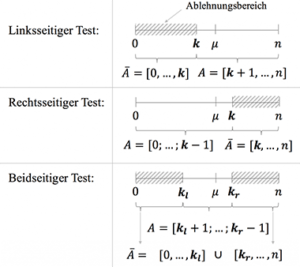Danger: Point number problem, do I round up or down?

In tests on both sides, one always rounds inwards, one also speaks of Rounds to the safe side, e.g. \$ k_l = 54.48 \$ and \$ k_r = 78.92 \$: \$ A = [55; 78] \$. With one-sided tests you have to pay attention to whether it is left or right-sided. For example, in a left-sided test with \$ k = 9.18 \$ or \$ k = 9.88 \$, the acceptance range \$ A = [10; n] \$ follows.

### Determine probabilities

When testing hypotheses, one would like to infer the population from a sample. For this reason, the sample should be selected as large as possible in order to be able to make a good statement. Since the test quantities are binomially distributed, we have to fall back on the binomial distribution to calculate probabilities.

In memory of:

\ begin {align *}
P (X \ leq k) = \ sum_ {i = 0} ^ k \ left (\ begin {array} {c} n \ i \ end {array} \ right) \ cdot p ^ i \ cdot (1- p) ^ {ni}
\ end {align *}

For large values ​​of \$ k \$ (typical for hypothesis tests) one should no longer calculate the probability by hand. Here you get to know two ways how you can easily determine the respective values.

### Read from the \$ F \$ table

A simple option, if you don't have a GTR / CAS at hand or just want to be faster, is to read it off from the \$ F \$ table. This table gives the cumulative probabilities of the binomial distribution from 0 to \$ k \$ for different \$ n \$ and \$ p \$ and should be given in the exam. There is also a \$ B \$ table of the binomial distribution that gives the probability of exactly \$ k \$ hits.

### Example:

If the probability of \$ P (X \ leq 2) \$ is searched for with \$ n = 10 \$ and \$ p = 0.1 \$, we first write it in the form \$ F (n; p; k) \$, i.e. here \$ F (10; 0,1; 2) \$ and then look for the appropriate \$ F \$ table for \$ n = 10 \$. You can see an excerpt from this table and how to get the correct value in the following illustration.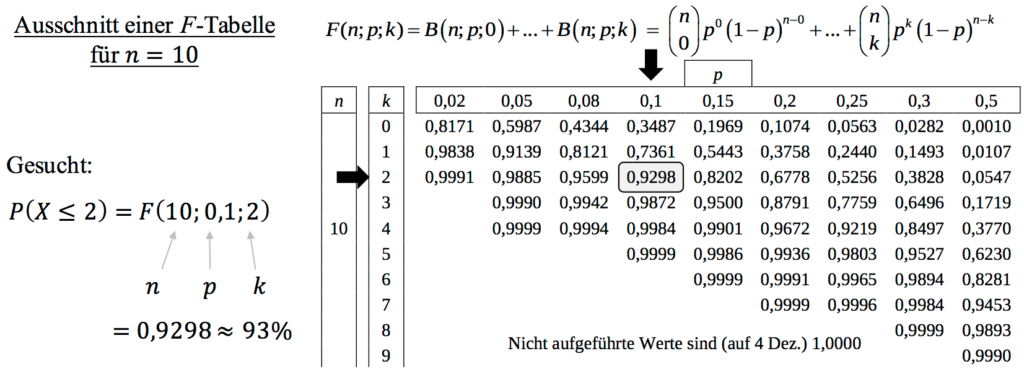Important: The searched probability must always be put in the form \$ \ leq \$! So before you can read the value for [/ latex] \ geq [/ latex] or \$> \$, you have to rewrite it, e.g .:

\ begin {align *}
P (X \ geq 2) = 1-P (X \ leq 1) \ quad \ textrm {or} \ quad P (X> 2) = 1-P (X \ leq 2)
\ end {align *}

Calculation with the GTR / CAS

If you work with the GTR / CAS, you only need the command: binomcdf \$ (n, p, k) \$! This command calculates the cumulative (summed up) probabilities of the binomial distribution and gives you the probability you are looking for. For the example above with \$ P (X \ leq 2) \$, \$ n = 10 \$ and \$ p = 0.1 \$ you get with the command \ texttt {binomcdf} \$ (10; 0.1; 2) \$ directly the searched value.

12 tasks with solutions PDF download ✓ preparing for Abiˈ20 ✓

1,99€

### Testing failure

A hypothesis can never be confirmed or refuted with absolute certainty, but only with a certain probability. This means that any decision we make based on a hypothesis can be wrong. Most of the time the mistake is that we came to a hasty conclusion or that we used incomplete information from our sample to make a general statement about the whole.

Let's think again of the example with the accused, who can be guilty or innocent. Convicting an innocent person is a type 1 error and acquitting a guilty person is a type 2 error.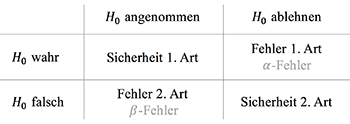The table above shows that 4 cases can occur:

a) We reject \$ H_0 \$, so accept \$ H_1 \$.

1. In reality \$ H_0 \$ is true: Here \$ H_0 \$ is wrongly rejected. This bug will Type 1 error called or \$ \ alpha \$ -error and describes the probability of error. The probability of making a Type I mistake is called Level of significance or probability of error \$ \ alpha \$.
2. In reality \$ H_1 \$ is true, so \$ H_0 \$ is wrong: Everything is okay, because \$ H_1 \$ is assumed and is actually true. One speaks of security type 2.

b) We assume \$ H_0 \$.

3. In reality \$ H_0 \$ is true: Everything is OK, because \$ H_0 \$ is assumed and is actually true. One speaks of type 1 security.
4. In reality, \$ H_1 \$ is true, so \$ H_0 \$ is false: Our guess is true (ie \$ H_1 \$, which we want to prove is true), but the test could not confirm it because we have \$ H_0 \$ accept. This error is reported as a Type 2 error or \$ \ beta \$ errors. We cannot control this probability; it depends on the type of test and the significance level \$ \ alpha \$.

Before carrying out the test, one has to commit to a significance level \$ \ alpha \$, which defines the maximum probability with which such a 1st type error can happen to us. The more certain we want to be with our decision, the lower this error probability has to be chosen. In the vast majority of cases, both in practice and in exams, this value is set as \$ \ alpha = 5 \$%.

In contrast to the type 1 error, the probability of the type 2 error is usually not easy to calculate. This can only be calculated if one assumes a different probability for the alternative hypothesis than for \$ H_0 \$.

In general, the smaller the probabilities of type 1 and type 2 errors, the better.

Important: You can never prove \$ H_0 \$, only \$ H_1 \$. For this reason, it is important to formulate the hypotheses the right way round: The case that you want to prove is included in the alternative hypothesis. The trial metaphor is a helpful donkey bridge to remember this practice.

### Example:

In a factory, one machine packs 100g of chocolate at a time.

• \$ H_0 \$: \$ \ mu = 100 \$ g (the machine works correctly)
• \$ H_1 \$: \$ \ mu \ neq 100 \$ g (the machine is not working correctly)

where \$ \ mu \$ is the average weight of the packages.

Let us now consider what errors can occur in our hypotheses.

1) In the event of a Type I error, the null hypothesis (\$ H_0 \$) is rejected despite the fact that it is true. For our example this would mean that although the machine would work correctly (hence \$ \ mu = 100 \$ g), we would find in our sample that the average weight is \$ \ mu \ neq 100 \$ g.

2)> In the case of an error of type 2, exactly the opposite happens: the machine does not work correctly, i.e. it does not pack an average weight of 100 g of chocolate, but our sample does not show this. According to her, the machine is working correctly.

Of course, we can also make the right decision based on our sample.

But what happens if our sample says that our null hypothesis is false because \$ \ mu \ neq is 100 \$ g? How does that affect the error when the average weight is actually 100g and when it is not 100g?

1) If \$ \ mu = 100 \$ g, the null hypothesis is true. If we reject them, we commit a mistake of type I.

2) If \$ \ mu \ neq is 100 \$ g, the null hypothesis is false. If we reject them, we will make the right decision.

Alpha / beta errors, 1st / 2nd type errors, testing, stochastics, math by Daniel Jung

12 tasks + solutions ✓ PDF available immediately ✓ preparing for Abiˈ21 ✓

New!

### Alternative test

As we have already learned in the overview, there is also an alternative test in addition to the one-sided and two-sided hypothesis tests. It is characteristic that it is not said that the null hypothesis has decreased, increased or changed, but that an alternative statement is given. To understand this, let's look at an example.

### Example:

Ms. Wanka says that 10% of all students study with videos. Daniel disagrees and claims that 30% of all students learn with videos. They decide that if at least 3 students study with videos, Daniel's hypothesis is correct.

In the afternoon Daniel drives to Remscheider City and asks 10 students whether they are learning with videos.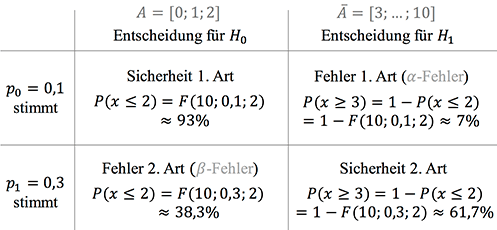Let's write down this information:

• Null hypothesis \$ H_0: \ p_0 = 0.1 \$ and alternative hypothesis \$ H_1: \ p_1 = 0.3 \$
• Test size \$ X \$: number of video learners; Sample: \$ n = \$ 10
• Acceptance range: \$ A = [0; 1; 2] \$, rejection range: \$ \ bar {A} = [3; 4; 5; 6; 7; 8; 9; 10] \$

Type 1 error is determined in the rejection area of ​​\$ H_0 \$ with a probability of \$ H_0 \$. There is a 7% chance that \$ H_0 \$ will be rejected even though \$ H_0 \$ is actually true.

The second type error is determined in the acceptance range of \$ H_0 \$ with the probability of \$ H_1 \$. There is a 38.3% probability that we will choose \$ H_0 \$, although \$ H_1 \$ is actually true.

12 tasks with solutions PDF download ✓ preparing for Abiˈ20 ✓

1,99€

### Hypothesis test with δ-rules

The \$ \ sigma \$ rules refer to the standard normal distribution. They indicate what percentage of the area under the bell curve is in the range of 1, 2 or 3 standard deviations to the left and right of the mean.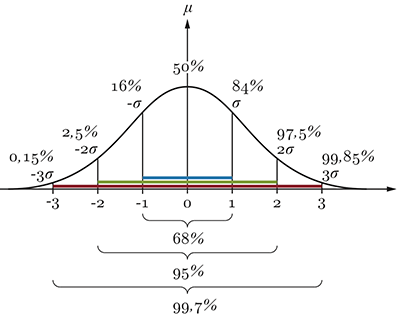Approximately 68% of the values ​​are within one standard deviation of the mean. Likewise, about 95% of the values ​​are within two deviations from the mean. And about 99.7% of the values ​​are within three standard deviations from the mean.

The standard deviation in the binomial distribution is calculated as follows:

\ begin {align *}
X \ sim B (n; p): \ quad \ sigma = \ sqrt {n \ cdot p \ cdot (1-p)}.
\ end {align *}

What do you get out of it?

If the scatter is large enough (Laplace condition: \$ \ sigma> 3 \$), the corresponding binomial distribution can usefully be approximated by the normal distribution and we can use the \$ \ sigma \$ environment for the rejection and acceptance area.

### Method:

• Make hypotheses \$ H_0 \$ and \$ H_1 \$.
• Decide whether you have a one-tailed or two-tailed hypothesis test.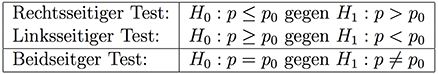• Calculate expected value: \$ \ mu = n \ cdot p \$
• Calculate standard deviation: \$ \ sigma = \ sqrt {n \ cdot p \ cdot (1-p)} \$
• Note the given probability of error.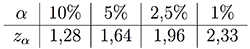• Establish decision-making rule: Determine acceptance (\$ A \$) and rejection areas (\$ \ overline {A} \$) with the \$ \ sigma \$ rule

\ begin {align *}
\ textrm {Two-sided test:} \ quad & A = \ left [\ mu - z _ {\ frac {\ alpha} {2}} \ cdot \ sigma; \ \ mu + z _ {\ frac {\ alpha} {2}} \ cdot \ sigma \ right] \
\ textrm {Left-sided test:} \ quad & \ overline {A} = \ left [0; \ \ mu - z _ {\ alpha} \ cdot \ sigma \ right] \
\ textrm {Right-sided test:} \ quad & \ overline {A} = \ left [\ mu + z _ {\ alpha} \ cdot \ sigma; \ n \ right]
\ end {align *}

In the decision rule, the rejection area and acceptance area are specified by specifying a significance level. The level of significance is the counter-probability to the certainty probability.

• Test decision based on the given sample \$ n \$.
• Calculate type 1 error and describe it in a factual context.
• Calculate type 2 error and describe it in a factual context.
12 tasks with solutions PDF download ✓ preparing for Abiˈ20 ✓

1,99€

### Double-sided hypothesis test:

The hypotheses are general in the case of the two-part or two-part hypothesis test

\ begin {align *}
H_0: ~ p = p_0; \ quad H_1: ~ p \ neq p_0.
\ end {align *}

Important: In a two-sided hypothesis test, both the left and right sides are considered for the rejection area. If a total of 10% is to be rejected, these percentages must be divided between the left and right areas. We can see what this means in the following example, if the significance level were \$ \ alpha = 5 \$%.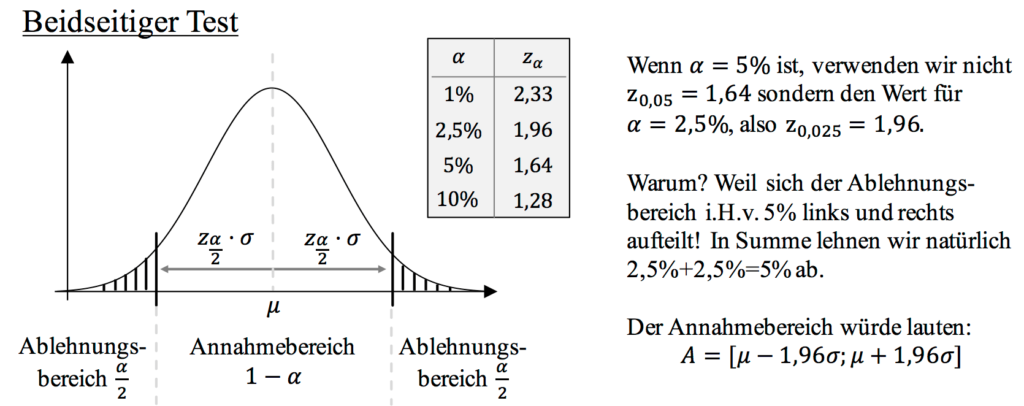### Example for a two-sided hypothesis test:

Football fans in a city are considered. 20% make up the fans of team A from B. We now take a sample of 158 soccer fans (level of significance: 5%).
The sample includes 43 football fans from team A and 115 from team B.

Can \$ H_0 \$ be discarded?

Also calculate the probability of type 1 and 2 errors if the actual percentage of football fans is 30%.

We work through the general procedure for this:

• First, hypotheses are made.
\ begin {align *}
H_0: ~ p = 0.2; ~ H_1: ~ p \ neq 0.2
\ end {align *}
• We recognize that this is a two-sided hypothesis test.
• Calculate expected value / point estimate:
\ begin {align *}
\ mu = n \ times p = 158 \ times 0.2 = 31.6
\ end {align *}
• Calculate the standard deviation and check the Laplace condition:
\ begin {align *}
\ sigma = \ sqrt {n \ cdot p \ cdot (1-p)} = \ sqrt {158 \ cdot 0.2 \ cdot (1-0.2)} = 5.03> 3 \ quad
\ end {align *}
• Note the given level of significance:
• \$ \ alpha = \$ 0.05 as the probability of error
• \$ 1- \ alpha = \$ 0.95 as a security probability
• \$ z _ {\ frac {\ alpha} {2}} \$, because a two-sided hypothesis test is available

We can read off this value in the quantiles of the standard normal distribution.

• Determine the acceptance and rejection area with the \$ \ sigma \$ rules:
\ begin {align *} A = \ left [\ mu - z _ {\ frac {\ alpha} {2}} \ cdot \ sigma, \ mu + z _ {\ frac {\ alpha} {2}} \ cdot \ sigma \ right] \ end {align *} with \$ \ sigma = 5.03 \$, \$ \ mu = 31.6 \$ and \$ z _ {\ frac {\ alpha} {2}} = 1.96 \$ follows \ begin { align *} A = \ left [31.6 - 1.96 \ cdot 5.03; \ 31.6 + 1.96 \ cdot 5.03 \ right] = \ left [22; 41 \ right]. \ End {align *} The rejection area is therefore \$ \ overline {A} = \ left [0; 21 \ right] \ cup \ left [42; 158 \ right] \$.
• Establish decision-making rule:
\$ H_0 \$ is discarded if no more than 21 or at least 42 people are football fans of team A. We can also visualize the decision rule graphically as follows.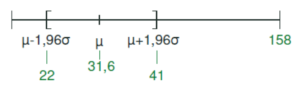• Test decision based on the given sample \$ n \$: The task states that there are 43 football fans in the sample, which means that this value does not fall into the acceptance range, but into the rejection range, which is located to the right and left of the acceptance range \$ [22 ; 41] \$ is located.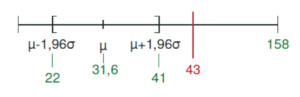The sample provides the result that \$ H_0 \$ can be discarded, since 43 football fans of team A are in the rejection area.
• Type 1 and Type 2 errors: Someone claims that the actual percentage of football fans is 30%, so this is an alternative test and we can calculate Type 1 and Type 2 errors. In the factual context, the type 1 error describes the probability that the hypothesis “20% of football fans are supporters of team A” will be rejected, even though it is correct. This was given in the exercise text and amounts to \$ \ alpha = 5 \$%. The 2nd type error means that a hypothesis is accepted although it is wrong. The calculation is done via \ begin {align *} P (22 \ leq X \ leq 41) = F (158; 0.3; 41) - F (158; 0.3; 21) \ end {align *} and can can be solved with the calculator or with the table. Since there is no table for \$ n = 158 \$ and the Laplace condition is fulfilled, we can approximate the binomial distribution by the normal distribution. Since there is an approximation from the binomial distribution (discrete) to the normal distribution (continuous), continuity corrections must be taken into account. It follows: \ begin {align *} P (a \ leq X \ leq b) & \ approx \ Phi \ left (\ frac {b + 0.5-np} {\ sqrt {np (1-p)}} \ right) - \ Phi \ left (\ frac {a-0,5-np} {\ sqrt {np (1-p)}} \ right) \ \ Rightarrow \ quad P (22 \ leq X \ leq 41) & \ approx \ Phi \ left (\ frac {41 + 0.5-47.4} {\ sqrt {5.76}} \ right) - \ Phi \ left (\ frac {22-0.5 -47.4} {\ sqrt {5.76}} \ right) \ \ & = \ Phi (-1.02) - \ Phi (-4.5) \ & = 1- \ Phi (1 , 02) - (1 - \ Phi (4,5)) \ & = 0.1539 \ approx 15.39 \% \ end {align *} 15.39% of the \$ H_0 \$ hypothesis is accepted, although this wrong is. This means that 15.39% believe that the proportion of soccer fans is 20%, although this is not the truth.
12 tasks with solutions PDF download ✓ preparing for Abiˈ20 ✓

1,99€

### One-sided hypothesis test

If a hypothesis test is only about whether the probability of an event has changed in one direction, then it is a one-sided hypothesis test.

If one suspects that the probability is smaller than previously assumed, one speaks of a left-hand hypothesis test or significance test.
If one suspects a greater probability of the event, one speaks of a right-hand significance test.

### Left-sided hypothesis test

In a left-hand hypothesis test, small values ​​of the random variables speak against the hypothesis, i.e. values ​​that are on the left of the number line or on the left of the expected value.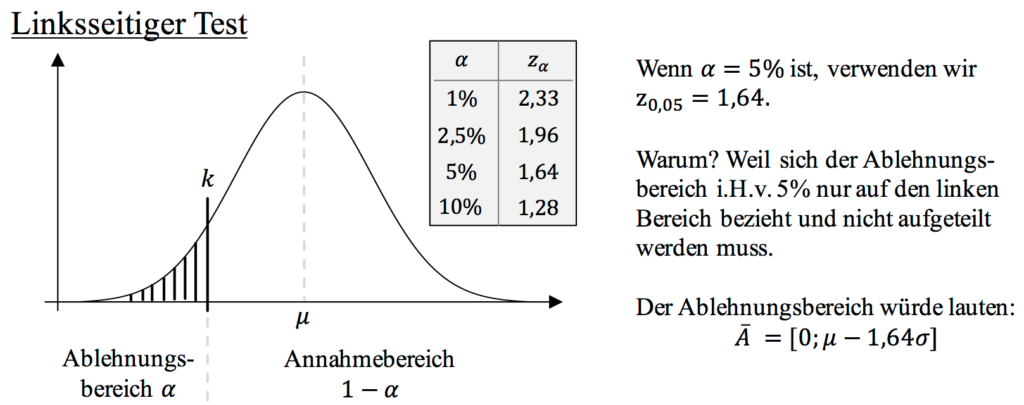### Example for the left-hand hypothesis test:

In the last election, a candidate received 40% of the votes cast. In order to check whether he has at least kept his share of the vote, a poll will be carried out some time before the next election. Out of 100 people, only 34 say they will vote for this candidate. Can one conclude from this with the 5% probability of error that the candidate's share of the vote has decreased?

• Make hypotheses:
\ begin {align *}
H_0: ~ p \ geq 0.4; ~ H_1: p <0.4
\ end {align *}
Read the text carefully! “Received at least 40% of the votes…” That would be a good thing and is therefore our null hypothesis.
• We recognize that this is a one-sided hypothesis test. Left-sided hypothesis test, as very small values ​​speak against \$ H_0 \$.
• Calculate expected value / point estimate:
\ begin {align *}
\ mu = n \ times p = 100 \ times 0.4 = 40
\ end {align *}
• Calculate the standard deviation and check the Laplace condition:
\ begin {align *} \ sigma = \ sqrt {n \ cdot p \ cdot (1-p)} = \ sqrt {100 \ cdot 0.4 \ cdot (1-0.4)} = 4.898> 3 \ quad \ end {align *}
• Note the significance level:
• \$ \ alpha = \$ 0.05 as the probability of error
• \$ 1- \ alpha = \$ 0.95 as a security probability
• \$ z _ {\ alpha} \$, because a one-sided hypothesis test is available

We can read off this value in the quantiles of the standard normal distribution.

• Determine the acceptance and rejection area with the \$ \ sigma \$ rules. In general:
\ begin {align *}
\ left [\ mu - z_ \ alpha \ cdot \ sigma \ right]
\ end {align *} We know the expected value and the standard deviation. From the quantiles of the standard normal distribution, \$ z_ \ alpha = 1.6449 \$. Insert and we get (rounded because on the left) the rejection and acceptance area: \ begin {align *} \ overline {A} = \ left [0; 31 \ right] \ quad \ textrm {and} \ quad A = \ left [32; 100 \ right] \ end {align *}
• Establish decision-making rule: \$ H_0 \$ is discarded if a maximum of 31 people vote for the candidate.
• Test decision based on the given sample \$ n \$: The question states that 34 people stated that they will choose the candidate. The result of the sample is that \$ H_0 \$ cannot be rejected, i.e. retained, since the value of the sample is in the acceptance range and not in the rejection range.
• Define type 1 error and then calculate / describe it in a factual context: The type 1 error means that a true hypothesis is rejected and this can be read off at the level of significance / probability of error, here 5%. This means that 5% of the \$ H_0 \$ hypothesis is wrongly rejected.

### Right-hand hypothesis test

In a right-hand hypothesis test, large values ​​of the random variables speak against the hypothesis, i.e. values ​​that are to the right of the number line or to the right of the expected value.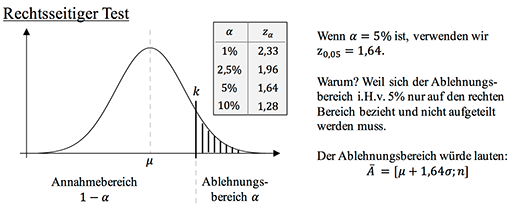12 tasks with solutions PDF download ✓ preparing for Abiˈ20 ✓

1,99€

### Example for the right-hand hypothesis test:

Bottles are filled and sealed in a lemonade factory. Not all bottles are properly closed. 100 bottles are checked every hour. Experience has shown that not more than 20% of the bottles are closed incorrectly. In a random sample, 25 bottles are found that are incorrectly closed.

1) How many incorrectly closed bottles are allowed to be found in the control? (Probability of error = 5%)
2) Calculate the type 2 error if actually 20% of the bottles are closed incorrectly.

To solve the task, we work through the known procedure:

• Make hypotheses:
\ begin {align *}
H_0: ~ p \ leq 0.2; ~ H_1: p> 0.2
\ end {align *}
Read the text carefully! “At most 20% of the bottles closed incorrectly…” is our null hypothesis.
• We recognize that this is a one-sided hypothesis test, since high values ​​speak against \$ H_0 \$.
• Calculate expected value / point estimate:
\ begin {align *}
\ mu = n \ times p = 100 \ times 0.2 = 20
\ end {align *}
• Calculate the standard deviation and check the Laplace condition:
\ begin {align *} \ sigma = \ sqrt {n \ times p \ times (1-p)} = \ sqrt {100 \ times 0.2 \ times (1-0.2)} = 4> 3 \ quad \ end {align *}
• Note the significance level:
• \$ \ alpha = \$ 0.05 as the probability of error
• \$ 1- \ alpha = \$ 0.95 as a security probability
• \$ z _ {\ alpha} \$, because a one-sided hypothesis test is available

We can read off this value in the quantiles of the standard normal distribution.

• Determine the acceptance and rejection area with the \$ \ sigma \$ rules. In general:
\ begin {align *}
\ left [\ mu + z_ \ alpha \ cdot \ sigma \ right]
\ end {align *} We know the expected value and the standard deviation. From the quantiles of the standard normal distribution, \$ z_ \ alpha = 1.6449 \$. Insert and we get (rounded up because on the right) the rejection and acceptance area: \ begin {align *} \ overline {A} = \ left [27; 100 \ right] \ quad \ textrm {and} \ quad A = \ left [0; 26 \ right]. \ End {align *}
• Set up decision rule: \$ H_0 \$ is discarded if more than 26 bottles are closed incorrectly.
• Test decision based on the given sample \$ n \$: The task states that 25 bottles were found in the sample that were incorrectly closed. This means that this value falls within the acceptance range. The result of the random sample is that \$ H_0 \$ cannot be discarded, i.e. retained, since the value of the random sample is in the acceptance range.
• Define type 1 error and calculate / describe it in the following in a factual context: Type 1 error means that a true hypothesis is rejected and that this can be read from the level of significance. This means that 5% of the \$ H_0 \$ hypothesis is rejected by mistake.
• Calculate type 2 error and describe it in a factual context: Type 2 error means that a hypothesis is accepted although it is in reality wrong. We calculate the error of the 2nd kind as usual: \ begin {align *} \ beta & = P (X \ leq 26) \ approx \ Phi \ left (\ frac {26 + 0.5-100 \ cdot 0.3} { \ sqrt {100 \ cdot 0.3 \ cdot (1-0.3)}} \ right) = \ Phi (-0.7637) \ & = 1- \ Phi (0.76) = 1-0, 7764 \ approx 22.36 \% \ end {align *} 22.36% of the test falls within the acceptance range, although more than 20% (namely 30%) incorrectly closed bottles can be found in the sample.
12 tasks with solutions PDF download ✓ preparing for Abiˈ20 ✓

1,99€

### Hypothesis test with reading from table

If, for whatever reason, you are not allowed to use the \$ \ sigma \$ rules, there is still the possibility of editing hypothesis tests with the help of tables. What you should already be able to do in this chapter is reading values ​​in the \$ F \$ table or, if you have to create these tables yourself with your GTR / CAS, how you can display them with the help of the binomcdf command. We will now only go into the pure reading from the table, since it is basically exactly the same as if you generated it beforehand with the calculator.

What is the difference to dealing with the \$ \ sigma \$ rules? When setting up the decision rule, i.e. how the acceptance and rejection area is determined. This is a bit more cumbersome here. The rest of the process is identical.

### Left-sided hypothesis test

Method:

1. Make hypotheses: \$ H_0: p \ geq p_0; \ H_1: p 2. Determination of the sample size \$ n \$ and the significance level \$ \ alpha \$
3. Determination of the rejection area: \$ \ overline {A} = [0; k] \$

The following must apply: \$ P (X \ leq k) \ leq \ alpha \$

4. Decision rule: If the sample size is in \$ \ overline {A} \$, \$ H_0 \$ is discarded, otherwise \$ H_0 \$ is retained.

example

It is claimed that at least 30% of students cheat on their math graduation exam. To test this thesis, 100 pupils from a school are interviewed. The level of significance is \$ \ alpha = 5 \$%. Derive a decision rule.

First we set up the hypotheses. The signal word in the task text is at leastso that we know directly that the hypotheses are as follows:

\ begin {align *}
H_0: p_0 \ geq 0.3; \ quad \ textrm {and} \ quad H_1: p_1 <0.3
\ end {align *}

From the exercise text we can also see that the sample size is \$ n = \$ 100 and the significance level is 5%. The probability of error is very important because it determines the critical value from when we accept the null hypothesis. For illustration, the function of the binomial distribution is shown again graphically.

It is now necessary to determine the critical value with the help of the appropriate \$ F \$ table (that was the one with the cumulative probabilities). The appropriate excerpt, which you have to look for yourself in the exam, is also shown.

It shows the \$ F \$ table for \$ n = 100 \$ for the probability \$ p = 0.3 \$.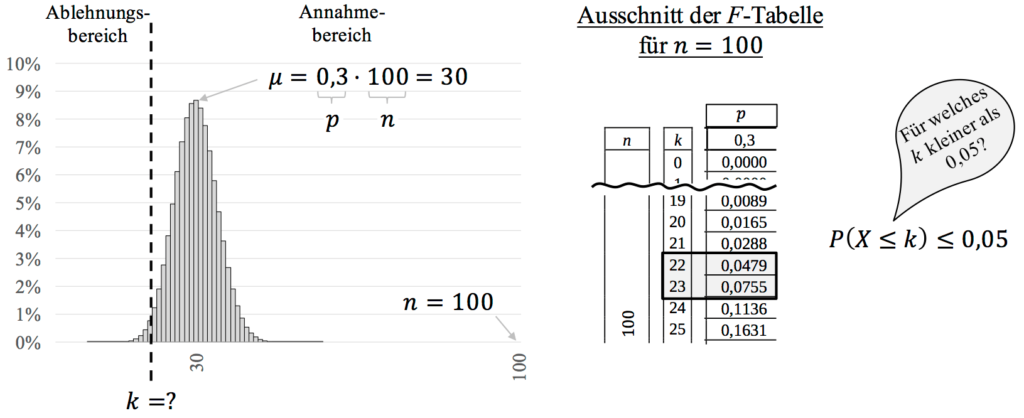The following condition applies to the rejection area: \$ P (X \ leq k) \ leq \ underbrace {0.05} _ {\ alpha} \$.

We are now looking for the probability in the table that is less than or equal to the probability of error in the amount of Is 0.05. We see that for \$ k = \$ 22 the value is just below 0.05, but at \$ k = \$ 23 the value is already exceeded.

You can then write in the exam:
\ begin {align *}
P (X \ leq k) = \ & F (100; ~ 0.3 ~; k) \ leq 0.05 \ notag \
& F (100; ~ 0.3 ~; 22) = 0.0479 \ leq 0.05 \ quad \ notag \
& F (100; ~ 0.3 ~; 23) = 0.0755> 0.05 \ quad \ notag
\ end {align *}

For the critical value we take the value that is just below 0.05. From this it follows for the rejection and acceptance area:

\ begin {align *}
\ overline {A} = [0; 22] \ quad \ textrm {and} \ quad A = [23; 100]
\ end {align *}

Remember to set up and formulate the decision-making rules! If, out of a total of 100 students, at most 22 say they are cheating, then the null hypothesis is rejected.
If a total of 23 or more students say they are cheating, then the \$ H_0 \$ hypothesis is maintained.

12 tasks with solutions PDF download ✓ preparing for Abiˈ20 ✓

1,99€

### Right-hand hypothesis test

Method:

1. Make hypotheses: \$ H_0: p \ leq p_0; \ H_1: p> p_0 \$
2. Determination of the sample size \$ n \$ and the significance level \$ \ alpha \$
3. Determination of the rejection area: \$ \ overline {A} = [k; n] \$

The following must apply: \$ P (X \ geq k) \ leq \ alpha \ Leftrightarrow 1- P (X \ leq k-1) \ leq \ alpha \$.

4. Decision rule: If the sample size is in \$ \ overline {A} \$, \$ H_0 \$ is discarded, otherwise \$ H_0 \$ is retained.

Example:

It is claimed that a maximum of 30% of students peck at their maths high school exam. To test this thesis, 100 pupils from a school are interviewed. The level of significance is \$ \ alpha = 5 \$%. Derive a decision rule.

First we set up the hypotheses. The signal word in the task text is at mostso that we know directly that the hypotheses are as follows:

\ begin {align *}
H_0: p_0 \ leq 0.3; \ quad \ textrm {and} \ quad H_1: p_1> 0.3
\ end {align *}

From the text of the exercise we can also see that the sample size is \$ n = \$ 100 and the significance level is 5%. The probability of error is very important because it determines the critical value from when we accept the null hypothesis.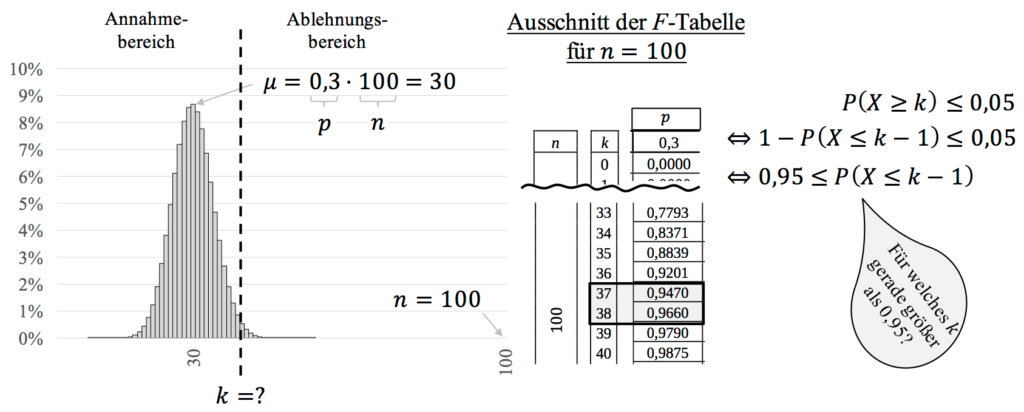For illustration, the function of the binomial distribution is shown again graphically. It is now necessary to determine the critical value with the help of the \$ F \$ table (that was the one with the cumulative probabilities). The appropriate excerpt, which you have to look for yourself in the exam, is also shown. This shows the \$ F \$ table for \$ n = 100 \$ and \$ p = 0.3 \$.

In contrast to the left-hand hypothesis test, we have to be careful when setting the rejection range, because the condition

\ begin {align *}
P (X \ geq k) \ leq \ underbrace {0.05} _ {\ alpha}
\ end {align *}

must first be rewritten with the opposite probability. Why? Because we only work with tables that sum the probabilities from 0 to \$ k \$ and not from \$ k \$ to \$ n \$.

Hence it follows from the condition:

\ begin {align *}
P (X \ geq k) \ leq 0.05 \ \ Leftrightarrow \ 1-P (X \ leq k-1) \ leq 0.05 \ \ Leftrightarrow \ 0.95 \ leq P (X \ leq k-1)
\ end {align *}

We look for the value in the corresponding \$ F \$ table that is greater than or equal to 0.95. For \$ k = \$ 37, we are just below 0.95, but for \$ k = \$ 38, the value of 0.95 is exceeded for the first time. That is the value with which we will determine the rejection area in a moment. Mathematically, you can write this down in the exam as follows:

\ begin {align *}
0.95 & \ leq P (X \ leq k-1) \
0.95 & \ leq F (100; ~ 0.3 ~; k -1) \
0.95 & \ leq F (100; ~ 0.3 ~; 37) = 0.947 \ quad \
0.95 & \ leq F (100; ~ 0.3 ~; \ underbrace {38} _ {= k-1}) = 0.966 \ quad \ notag
\ end {align *}

For the critical value we take the value that is just above 0.95. Now we have to be careful, because the critical value is \$ k-1 = 38 \$, so \$ k = 39 \$.

This means that for the rejection and acceptance area:

\ begin {align *}
\ overline {A} = [39; 100] \ quad \ textrm {and} \ quad A = [0; 38].
\ end {align *}

Remember to set up and formulate the decision-making rules! If at least 39 of a total of 100 students say they are cheating, then the null hypothesis is rejected.
If a total of 38 or fewer students say they are cheating, then we can confirm the \$ H_0 \$ hypothesis.

12 tasks with solutions PDF download ✓ preparing for Abiˈ20 ✓

1,99€

### Double-sided hypothesis test

Method:

1. Make hypotheses: \$ H_0: p = p_0; \ H_1: p \ neq p_0 \$
2. Determination of the sample size \$ n \$ and the significance level \$ \ alpha \$
3. Determination of the rejection area: \$ \ overline {A} = [0; k_l] \ cup [k_r; n] \$

The following must apply: \$ P (X \ leq k_l) \ leq \ alpha / 2 \$ and \$ P (X \ geq k_r) \ leq \ alpha / 2 \$.

4. Decision rule: If the sample size is in \$ \ overline {A} \$, \$ H_0 \$ is discarded, otherwise \$ H_0 \$ is retained.

Example:

It is claimed that the rate that 30% of students cheat on their maths exam has changed. To test this thesis, 100 pupils from a school are interviewed. The level of significance is \$ \ alpha = 5 \$%. Derive a decision rule.

First we set up the hypotheses. The signal word in the task text is changedso that we know directly that the hypotheses are as follows:

\ begin {align}
H_0: p_0 = 0.3 \ quad \ textrm {and} \ quad H_1: p_1 \ neq 0.3 \ notag
\ end {align}

The rest of the procedures are the same as for the one-sided test. We practically do a left and right-sided test in one. The only difference: We have to be careful with the significance level \$ \ alpha \$, as it is divided between the two rejection areas. The distribution is shown in the following figure with the corresponding excerpts from the \$ F \$ table.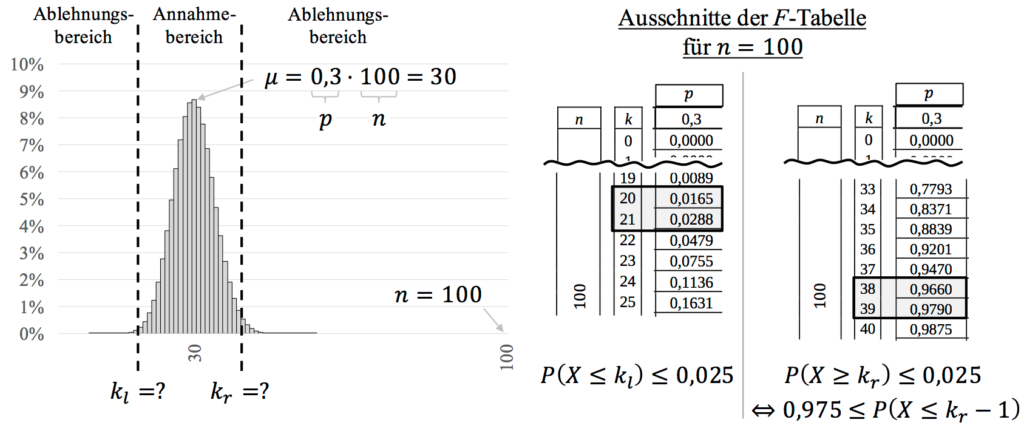From the conditions it follows for the critical values ​​of the rejection area:

\ begin {align *}
F (100; ~ 0.3 ~; k_l) & \ leq 0.025 \ notag \
F (100; ~ 0.3 ~; 20) & = 0.0165 \ leq 0.025 \ quad \ notag \
F (100; ~ 0.3 ~; 21) & = 0.0288> 0.025 \ quad \ notag
\ end {align *}

\ begin {align *}
0.975 & \ leq F (100; ~ 0.3 ~; k_r-1) \ notag \
0.975 & \ leq F (100; ~ 0.3 ~; 38) = 0.966 \ quad \ notag \
0.975 & \ leq F (100; ~ 0.3 ~; \ underbrace {39} _ {k_r-1}) = 0.979 \ quad \ notag
\ end {align *}

For the critical value \$ k_r \$ we take the value that is just above 0.975. Now we have to be careful, because the critical value is \$ k_r-1 = 39 \$, so \$ k_r = 40 \$. This means that for the rejection and acceptance area:

\ begin {align *}
\ overline {A} = [0; 20] \ cup [40; 100] \ quad \ textrm {and} \ quad A = [21; 39]
\ end {align *}

If, out of a total of 100 students, a maximum of 20 and at least 40 indicate that they are pecking at the Abi exam, then the hypothesis would be rejected. If in the range 21 to 39 students indicate that they are cheating in the exam, then the hypothesis is confirmed and we stick with it.

12 tasks with solutions PDF download ✓ preparing for Abiˈ20 ✓

1,99€